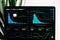Photo by Luke Chesser on Unsplash

# Time Series Data Analysis using Datalab, Pandas & Prophet

A time series is a series of data points indexed (or listed or graphed) in time order. Most commonly, a time series is a sequence taken at successive equally spaced points in time. Thus it is a sequence of discrete-time data. Examples of time series are heights of ocean tides, counts of sunspots, and the daily closing value of the Dow Jones Industrial Average.

There are a number of tools available to analyse time series data, plot and generate insights. This post outlines my experience with one such data analysis tool called Pandas. Pandas is a software library for Python programming language, which offers data structures and operations for analysing time series.

## Setup

The first tool of choice to use Pandas framework was Jupyter (acronym of Julia, Python and R, the three core languages supported) notebook. Jupyter can be run using Anaconda framework or using pip. Google Cloud provides a hosted version of Jupyter called Datalab. Datalab is what I have used for my prototype. Simply start a cloud shell and run the command:

`datalab create <vm-name>`

A new VM is created, launched and a port forwarding is also created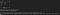Figure 1: Launching Datalab

## Reading, parsing and merging CSV files using Pandas

The source files are in CSV file format, with one for each month. The files were uploaded into Google Cloud Storage for easy analysis. Use the below library to access GCS. The below commands retrieves the CSV file ‘data-export-site-2018–09-Sep18–5m.csv’ from the bucket ‘demo-bucket-horizonx’ and returns the path.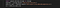Figure 2: Read CSV from Pandas

When running Python in Jupyter, IPython is used. IPython is a rich toolkit which allows running python interactively. IPython provides ‘magic commands’, very similar to command line tools which can be run within the shell. Datalab provides magic commands to easily access resources within Google Cloud like BigQuery, Google Cloud Storage, BigTable etc.

## Pandas DataFrame

To access GCS, Datalab provides a magic command called “gcs”. It reads the CSV from the GCS URI into a variable data. This is, in turn, converted to a Pandas DataFrame object using the function read_csv. In the below snippet, df_sept is a Pandas DataFrame. The procedure is repeated for all the months available.Figure 3: Parse CSV into Pandas Dataframe

If you have a compressed CSV file, Pandas can read that as well into a DataFrame

`df = pd.read_csv(BytesIO(data),compression='gzip',usecols=['col1','col2','col3','date_time_utc'])`

DataFrame info function will spit out interesting information about the frame.

`<class 'pandas.core.frame.DataFrame'>RangeIndex: 310370 entries, 0 to 310369Data columns (total 2 columns):Date_Time     310370 non-null datetime64[ns]Energy (J)    310370 non-null int64dtypes: datetime64[ns](1), int64(1)memory usage: 4.7 MB`

Once we have individual frames, the next step is to merge all of them together.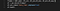Figure 4: Merge Dataframes

The variable frames is an array of dataframes, and Pandas function concat merges all of them into a single dataframe. Next line sets the column Date_Time as an index. The head function returns top 5 records by default.

If you are running Jupyter and want to access files from Google Cloud Storage, the library gcsfs can be used: https://github.com/dask/gcsfs

The DataFrame object provides an easy way to calculate the mathematical statistics functions.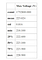Figure 5: Mean, median and mode
`data.dropna().describe()`

dropna function drops empty fields and describe function calculates the standard mathematical functions.

Plotting

Plotting is an important capability in Jupyter notebook. There are a number of frameworks like matplotlib, Seaborn, mpld3, bokeh, Altair and others. matplotlib is the de-facto standard. Seaborn is based on matplotlib and makes the matplotlib plots richer. Below is a plot using seaborn, which shows a summary of three columns averaged to week.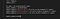Figure 6: Seaborn Plot Code

The resulting plot looks like this: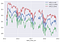Figure 7: Seaborn Plot

Another example using matplotlib, showing two plots overlayed and gives an indication of anomalies.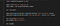Figure 8: Matplotlib plot code

The resulting plot: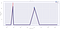Figure 9: Matplotlib plot

## Facebook Prophet for prediction

Prophet is a forecasting tool for Python and R. It always takes a DataFrame with two columns ‘ds’ (timestamp) and ‘y’ and provides two methods fit and predict.

The below code snippet demonstrates how to resample the Pandas DataFrame to be used with Prophet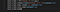Figure 10: DataFrame for Prophet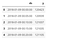Figure 11: Resampled DataFrame

Resample DataFrame for input into Prophet

Next step is to create a Prophet object and fit the DataFrame using the object

`from fbprophet import Prophetm = Prophet()m.fit(input_frame)`

Next step will create a dataframe with future dates (6 months)

`future = m.make_future_dataframe(periods=182)`

Run a prediction using the framework

`forecast = m.predict(future)forecast[['ds', 'yhat', 'yhat_lower', 'yhat_upper']].tail()`

Gives the below output: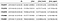Figure 12: Predictions

Now using this, we can simply plot the prediction or plot the seasonality component.

`fig2 = m.plot_components(forecast)`

This gives the following plot: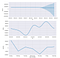Figure 11: Seasonality Component

## Conclusion

Pandas is a rich framework which fills the gap Python has in data analysis. Easy to use without much programming, it allows easy filtering, slicing and plotting of data as series or data frames.

Jupyter is a great interactive tool to explore, transform, visualise and share the analysis. It has a very rich ecosystem of modules to explore data across various sources and optimise machine learning models for deployments.

Written by

## HorizonX

#### We’re a team of passionate, expert and customer-obsessed practitioners, focusing on innovation and invention on our customer’s behalf.

Welcome to a place where words matter. On Medium, smart voices and original ideas take center stage - with no ads in sight. Watch
Follow all the topics you care about, and we’ll deliver the best stories for you to your homepage and inbox. Explore
Get unlimited access to the best stories on Medium — and support writers while you’re at it. Just \$5/month. Upgrade